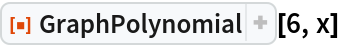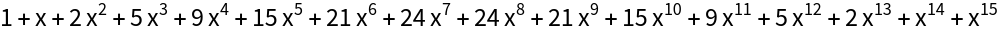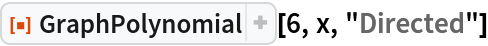Function Repository Resource:

# GraphPolynomial

Get the polynomial with coefficients giving the number of nonisomorphic graphs for a given number of vertices

Contributed by: Wolfram Staff (original content Sriram Pemmaraju and Steven Skienna)
 ResourceFunction["GraphPolynomial"][n,x] computes the polynomial in x for which the coefficient of xm is the number of nonisomorphic graphs with n vertices and m edges. ResourceFunction["GraphPolynomial"][n,x,"Directed"] computes the polynomial with coefficients that count nonisomorphic directed graphs.

## Examples

### Basic Examples (2)

Compute a graph polynomial:

 In:=Out=A graph polynomial for a directed graph:

 In:=Out=Enrique Zeleny

## Version History

• 1.0.0 – 24 August 2020